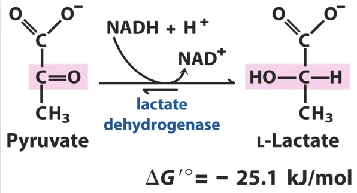## BCT105

Anjan Kr Dasgupta
Lecture 3

## Phenomenological Equations

Let us recall the linear phenomenological equation as we discussed before

$J_i=\Sigma L_{ij} X_j$
Few important attributes of the above are:
- J and X are fluxes and forces (efffects and causes)
- In chemical systems X is sign changed free energy
- In transport or diffusion systems X is electrochemical potential gradient

## Some thermodynamic questions

• How do we thermodynamically define coupled systems?
• Can the phenomenological coupling coefficients (whose symmetry is the Onsager relation) be valid in biological systems that normally shows a non-linear behavior
• In other words, will $L_{ij}=L_{ji}$ be valid for a system as complex as respiring mitochondria or photophosphorylating chloroplsts?

## Insights in Coupling

• Conventionally two chemical pathways are said to be coupled if there is a common intermediate
• If there is no common intermediate coupling of two reactions pathways will be difficult to conceive in the stoichiometric sense.
• However thermodynamic definition of coupling is still possible as long as the coupling coefficients (off-diagonal elements of the L matrix) are non-vanishing

## Fluxes in Oxidative Phosphorolyation

In oxidative phosphorylation we have
- Flux $J_P$ the rate of ATP synthesis
- Flux $J_o$ the rate of oxygen uptake

## Forces in Oxidative Phosphorylation

• Force $-\Delta G_p$ free energy driving the ATP synthesis
• Force $-\Delta G_o$ representing the free energy of electron transport that drives the phosphorylation

## Can we write the following?

$J_o= L_{OO}(-\Delta G_o)+ L_{OP}(-\Delta G_P)$ $J_P= L_{PO}(-\Delta G_o)+ L_{PP}(-\Delta G_P)$ We know that oxidative process and phosphorylation process are indeed coupled.But how can we have in absence of any common high energy intermediate, $L_{OP} \ne 0,L_{PO}\ne 0$Secondly, will $L_{OP}=L_{PO}$ be satisfied? If so, it will be a proof for validity of Onsager reciprocal relation in biological systems.

## New thermodynamic dimension by Peter Mitchell

• Chemical view: Coupling can exist only in presence of a common intermediate
• Experiment : There are solid experimental ground to ensure that coupling exists - but no high energy intermediate exists that the shared by the coupling pair
• Mitchell showed that protons , rather gradient o protons replaces the common intermediate.

## A list of Redox Reactions

$oxidant + e^{-} \rightarrow reductant$

Oxidant Reductant n E´0 (V)
Succinate + CO2 alpha-Ketoglutarate 2 - 0.67
Acetate Acetaldehyde 2 - 0.60
Ferredoxin (ox) Ferredoxin (red) 1 - 0.43
2 H+ H2 2 - 0.42

## Continued

Oxidant Reductant n E´0 (V)
Lipoate (oxidized) Lipoate (reduced) 2 - 0.29
Glutathione (ox) Glutathione (red) 2 - 0.23
Acetaldehyde Ethanol 2 - 0.20
Pyruvate Lactate 2 - 0.19
Fumarate Succinate 2 0.03

## Continued

Oxidant Reductant n E´0 (V)
Cytochrome b (+3) Cytochrome b (+2) 1 0.07
Dehydroascorbate Ascorbate 2 0.08
Ubiquinone (ox) Ubiquinone (red) 2 0.10
Cytochrome c (+3) Cytochrome c (+2) 1 0.22
Fe (+3) Fe (+2) 1 0.77
½ O2+ 2 H+ H2O 2 0.82

## Redox Potentials and Free-Energy Changes

The relation used to translate redox potential to free energy is: $\Delta G^{o'} = nF E_o'$ - n is the number of electrons transferred
- F is a proportionality constant called the faraday
- F=23.06 kcal mol-1 V-1
- F= 96.48 kJ mol-1 V-1
- Redox potential is in volts, free energy is in kilocalories or kilojoules per mole.

## Let us audit the energyscale=0.1

Oxidant Reductant n E´0 (V)
Pyruvate Lactate 2 - 0.19

## Stepwise

For the reaction involving conversion of the nicotinamide adenine dinucleotide from its its reduced to oxidized form: $NADH \rightarrow NAD+ H^+ + 2e^- (\Delta G_1)$ the free energy is given by

##  Free energy in KJ /mole = -61.75

For the pyruvate $pyruvate + 2H^+ + 2e^- \rightarrow Lactate (\Delta G_2)$

##  Free energy in KJ /mole = 36.66

## The Overall Free Energy

$\Delta G_1 + \Delta G_2$ (KJ/Mole=)

##  -25.0848

## Driving and driven reactions

2nd law demands $J1X1+J2X2>0$ If J1X1 <0 , then '1' is driven and 2 is dricing reaction and vice versa. - The driving force in this reaction is given by oxidation of NADH - The driven reaction in this case is the pyruvate to lactate conversion ## A few Notes on Fluxes and Forces

One can see that in this case $\Delta G_1 <0 , \Delta G2>0$ implying $X_1>0 , X_2<0$

## Efficiency

In the phenomenological context we can define the two fluxes as J1 and J2 (assuming both are positive).

The non-equilibrium efficiency of the process is given by : $\eta = -\frac{J_1 (-\Delta G_1 )}{J_2 (-\Delta G_2)}$ One can easiliy prove that second law of thermodynamics implies that $\eta \le 1$.

## Showing 1.14-Volt Potential Difference Between NADH and O2

This difference drives Electron Transport Through the Chain and Favors the Formation of a Proton Gradient.In this case let us take the two redox couples namely oxygen /water and NADH/NAD having potentials -0.32 and +0.82 V. The overall all direction will be towards the terminal acceptor oxygen and the magic number 1.14 V is obtained. ## Data Bases and links

Pathway Database

acidophillic

pH and photosynthesis